# Weighted Average Overtime Calculation Examples

## Overview

Updated 09/23/2022

Weighted Average Overtime Calculation [WAOT Calc].

## The following are the steps to determine the Weighted Average Overtime Rate:

1. Determine the Total Hours by adding all hours from all Assignments/Placements for the talent.
2. Determine the Total Regular Pay amount by using the total hours for each Assignment/Placement multiplied by the Assignment/Placement’s regular rate
[For each Assignment/Placement ((REG hrs + OT Hrs + DT hours) x REG rate) + (Non-Discretionary Bonuses)] and add all Assignment/Placement amounts together.
3. Determine the Calculated “Regular Rate” by taking the Total Regular Pay divided by the Total Hours.
4. Determine the Weighted Average Overtime Premiums
1. Weighted OT Premium by dividing the Calculated Regular Rate by 50%.
2. Weighted DT Premium (if applicable) by dividing the Calculated Regular Rate by 100%.
5. Determine the Weighted Average Overtime and Doubletime Rates by adding the Assignment/Placement’s Regular Rate plus the Weighted Average Premium.
6. Apply the Weighted Average Overtime and Doubletime Rates, in Classic Time Entry, to the talent.

## Scenario 1

### The employee has two Assignments/Placements in the same supplier

Employee Madison works a total of 65 hours in two Assignments/Placements.

• Assignment ID 182098 was entered as 50 hours REG at a Pay Rate of \$10 and OT Plan = US Fed OT Plan [40 Max REG hours].

As of the result of entering REG hours for 50 Pay Units, the system reallocates the REG hours to 40 Pay Units and OT hours to 10 Pay Units.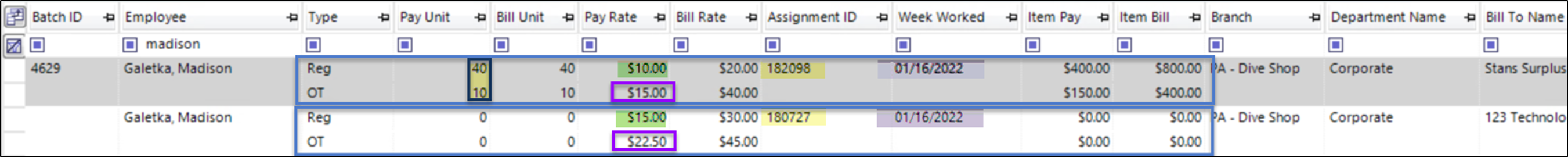The break-out from 50 hours to REG of 40hrs, and OT of 10hrs, is an example of what to we refer to as “Normalizing” the REG hours for the purpose of calculating overtime.

• Assignment ID 180727 was entered as 15 hours REG at a Pay Rate of \$15 and OT Plan = US Fed OT Plan [40 Max REG hours].

Since the Assignments/Placements are in the same Supplier [FEIN], the system takes into consideration the REG hours already entered of 40 Pay Units. It then takes the entered REG hours of 15 Pay Units and allocates them to OT hours of 15 Pay Units.

Additionally, because each Assignment/Placement has a unique rate of pay, the system then calculates the Weighted Average Overtime Rate for both Assignments. First Assignment 180727 is updated.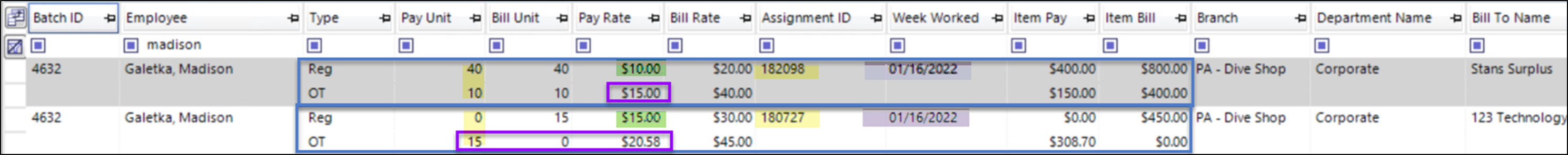However, the Weighted Average Overtime Rate for Assignment 182098 will not update until the screen is Refreshed.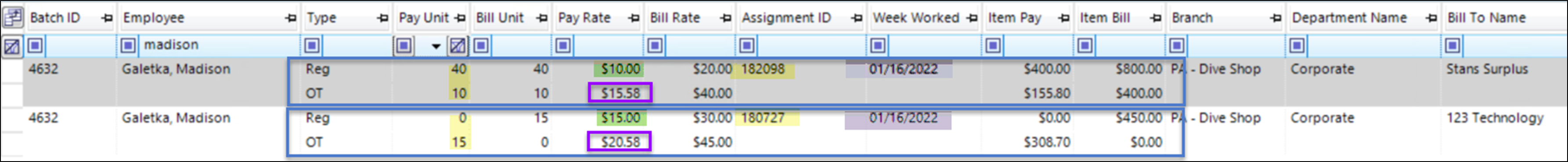See the details below to see how the Weighted Average Overtime Rates, that are outlined in blue, were determined.

How the Weighted Average Overtime Calculation determined the Weighted Average Overtime Rates:

1. Determine the Total Hours:
1. (Assignment ID 182098 REG+OT) + (Assignment ID 180727 REG+OT) = (40 + 10) + (15) = 65 hours
2. Determine the Total Regular Pay:
1. Total the Regular pay from applicable transaction types for each Assignment/Placement (Assignment ID 182098 Total Hours x Actual Regular Rate) + (Assignment ID 180727 Total Hours x Actual Regular Rate) + Non-Discretionary Bonus amount = (50 x \$10) + (15 x \$15) + 0 = (\$500) + (\$225) = \$725
3. Calculate the Calculated “Regular Rate”:
1. Total Regular Pay / Total Hours
\$725 / 65 = \$11.153846
4. Determine the Weighted Average Overtime Premium
1. Calculated Regular Rate x 50%
\$11.153846 x .5 = 5.58
5. Determine the Weighted Average Overtime Rate
1. Actual Regular Rate + Weighted Average Overtime Premium
2. Assignment ID 182098: \$10.00 + \$5.58 = \$15.58
3. Assignment ID 180727: \$15.00 + \$5.58 = \$20.58

## Scenario 2

### The employee already has a prior transaction that was closed and processed for the same Week Worked.

Employee Madison works a total of 55 hours in two Assignments/Placements.

• Assignment ID 182098 was entered as 45 hours REG at a Pay Rate of \$10 and OT Plan = US Fed OT Plan [40 Max REG hours].

As of the result of entering REG hours for 45 Pay Units, the system reallocates the REG hours to 40 Pay Units and OT hours to 5 Pay Units.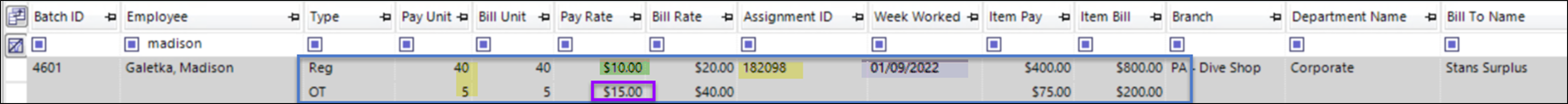The Time Entry transaction for Assignment ID 182098 is CLOSED and processed through payroll.

• Then, for Assignment ID 180727 there is an entry of 10 hours REG at a Pay Rate of \$15 and OT Plan = US Fed OT Plan [40 Max REG hours].

Since the Assignments/Placements are in the same Supplier [FEIN], the system takes into consideration the REG hours already entered of 40 Pay Units. It then takes the entered REG hours of 10 Pay Units and allocates them to OT hours of 10 Pay Units.

Additionally, because each Assignment/Placement has a unique rate of pay, the system then calculates the Weighted Average Overtime Rate for Assignment 180727.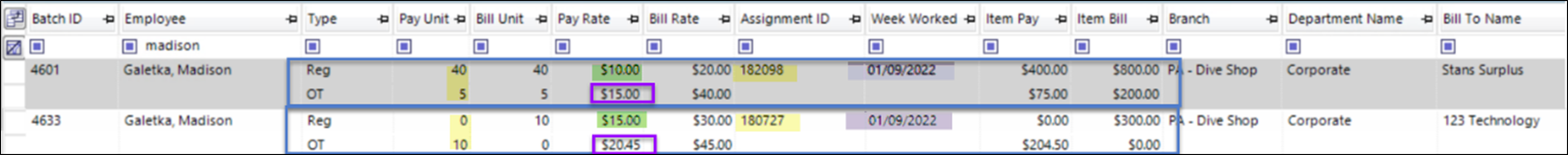However, the Weighted Average Overtime Rate for Assignment 182098 will not update as the transaction was previously closed. It is up to the customer to make adjustments for previous closed transaction.

See the details below to see how the Weighted Average Overtime Rates, that are outlined in blue, were determined.

How the Weighted Average Overtime Calculation determined the Weighted Average Overtime Rates:

1. Determine the Total Hours:
1. (Assignment ID 182098 REG+OT) + (Assignment ID 180727 REG+OT) = (40 + 5) + (10) = 55 hours
2. Determine the Total Regular Pay:
1. Total the Regular pay from applicable transaction types for each Assignment/Placement (Assignment ID 182098 Total Hours x Actual Regular Rate) + (Assignment ID 180727 Total Hours x Actual Regular Rate) + Non-Discretionary Bonus amount = (45 x \$10) + (10 x \$15) + 0 (\$450) + (\$150) = \$600
3. Calculate the Calculated “Regular Rate”:
1. Total Regular Pay / Total Hours
\$600 / 55 = \$10.90909
4. Determine the Weighted Average Overtime Premium
1. Calculated Regular Rate x 50%
\$10.90909 x .5 = 5.45
5.  Determine the Weighted Average Overtime Rate
1. Actual Regular Rate + Weighted Average Overtime Premium
2. Assignment ID 180727: \$15.00 + \$5.45 = \$20.45
3. [Assignment ID 182098 the OT Rate is not update as it was previously closed]

## Scenario 3

### The employee has a Non-Discretionary Bonus

Non-Discretionary Bonuses are included in the calculation of overtime. The value of the bonus will increase the amount of earnings when determining the Overtime and Doubletime rates.

Employee Madison works 50 hours, but then also receives a non-discretionary bonus of \$60.

• Assignment ID 182098 was entered as 50 hours REG at a Pay Rate of \$10 and OT Plan = US Fed OT Plan [40 Max REG hours].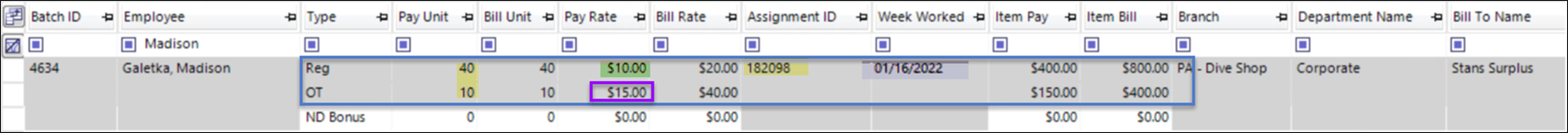As of the result of entering REG hours for 50 Pay Units, the system reallocates the REG hours to 40 Pay Units and OT hours to 10 Pay Units.

• Then, the employee then also receives a Non-Discretionary Bonus of \$60.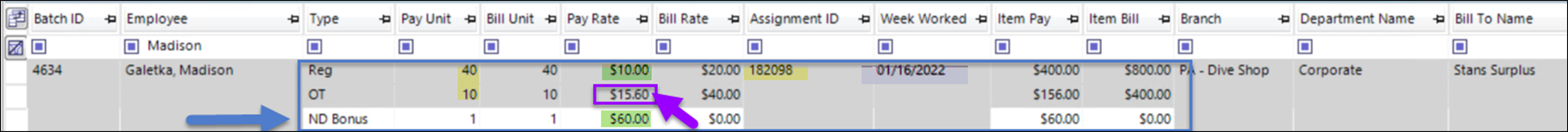As of the result of entering the Non-Discretionary Bonus, the system will calculate out the Weighted Average Overtime Premium, then determine the Weighted Average Overtime Rate

How the Weighted Average Overtime Calculation determined the Weighted Average Overtime Rates:

1. Determine the Total Hours:
1. (Assignment ID 182098 REG+OT) =
(40 + 10) + 0 = 50 hours
2. Determine the Total Regular Pay:
1. Total the Regular pay from applicable transaction types
(Assignment ID 182098 Total Hours x Actual Regular Rate) + Non-Discretionary Bonus amount = (50 x \$10) + \$60 = \$500 + \$60 [ND Bonus] = \$560
3. Calculate the Calculated “Regular Rate”:
1. Total Regular Pay / Total Hours
\$560 / 50 = \$11.20
4. Determine the Weighted Average Overtime Premium
1. Calculated Regular Rate x 50%
\$11.20 x .5 = 5.6
5. Determine the Weighted Average Overtime Rate
1. Actual Regular Rate + Weighted Average Overtime Premium
\$10.00 + \$5.60 = \$15.60